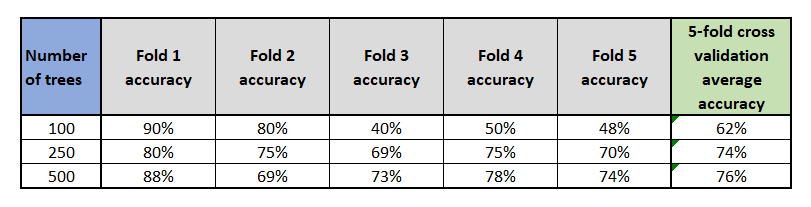Search
•Ekta Aggarwal

# K-fold cross validation explanation made easy!

Performance of many of the algorithms like K-Nearest Neighbours (KNN), Random Forests,

Decision Trees etc. are highly dependent upon the value of the parameters being passed on to

these algorithms. For instance, the value of k in KNN can extremely impact the results. Not

only that, there exists possibility of having a extremely good training set which is leading to

high accuracy, but the model performs poorly on test set, leading to overfitting. Thus, for this

we leverage the approach of K-fold cross validation where we divide the training set into K

groups which are mutually exclusive but completely exhaustive and are approximately equal

sized. K-fold cross validation is used to find the best value of hyperparameters.

### Algorithm Explained

Suppose we have 1000 data points, and we want to run K-fold cross validation for K = 5. Thus we will divide our data in 5 equal parts, where each of the parts are not overlapping with each other. Thus, in this way each part will have 200 points each. Let us call the folds as F1, F2, F3, F4 and, F5.

Let us keep the first fold of 200 points aside (F1) and we will build the model on F2, F3, F4, and F5. Now, we will use F1 to get the accuracy. Let us call this accuracy as "Acc1".

Similarly, we will keep F2 aside, and then build the same model with same hyperparameters on F1, F3, F4, and F5. Now, we will use F2 to get the accuracy. Let us call this accuracy as "Acc2".

Similarly we can get Acc1, Acc2, Acc3, Acc4, Acc5 . An average of these accuracies is calculated.

Figure below illustrates the procedure for K-fold cross validation for K=5.### Utility in finding best hyperparameter

Suppose we want to test various values of number of trees (say, ntree) such as 100, 250 and 500 in Random Forests.

We take the value of ntree = 100 and treat the first fold as a validation set and build the model on

K-1 folds . The accuracy is computed for first fold (say Acc1). Same is repeated for all the folds for ntree = 100. Ultimately, a final average accuracy is computed. Similarly, average accuracy for all the folds are computed for ntree = 250 and 500. The value of ntree which leads to highest performance is considered as the best estimate.

### An example

Following example best illustrates the application of K - Fold Cross validation:

In the table below, 5-fold cross validation was run for each of the ntree = 100,250 and 500. Then, we calculated the average accuracies for each of the parameters. We can see that with 100 trees, our 5-fold cross validation led to 90% accuracy in first fold, but bad accuracies on Folds 3, 4, and 5, leading to least average accuracy of 62%.

Fold ntree = 250 and 500, our model led to good accuracies in all the folds, but ntree = 500 led to highest average accuracy. Thus, we would prefer 500 trees for our model.Tags:

See All